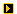YOU CAN CODE!With The Case Of UCanCode.net  Release The Power OF  Visual C++ ! Home |  Products | Purchase |  Support | DownloadsDownload EvaluationPricing & Purchase?E-XD++Visual C++/ MFC ProductsOverviewElectronic Form SolutionVisualization & HMI SolutionPower system HMI SolutionCAD Drawing and Printing SolutionBar code labeling SolutionWorkflow SolutionCoal industry HMI SolutionInstrumentation Gauge SolutionReport Printing SolutionGraphical modeling SolutionGIS mapping solutionVisio graphics solutionIndustrial control SCADA &HMI SolutionBPM business process SolutionIndustrial monitoring SolutionFlowchart and diagramming SolutionOrganization Diagram SolutionGraphic editor Source CodeUML drawing editor Source CodeMap Diagramming SolutionArchitectural Graphic Drawing SolutionRequest EvaluationActiveX COM ProductsOverviewTechnical Support# Get Ready to Unleash the Power of UCanCode .NET

UCanCode Software focuses on general application software development. We provide complete solution for developers. No matter you want to develop a simple database workflow application, or an large flow/diagram based system, our product will provide a complete solution for you. Our product had been used by hundreds of top companies around the world!

"100% source code provided! Free you from not daring to use components because of unable to master the key technology of components!"

CAM simulator Sample with VC++ MFC Source Code

By Diego Andrade## Introduction

After 6 months of work, here is finally my first MFC program release. This program is a CAM simulator. I am not a software engineer, so please forgive me for errors. If you have questions please let me know. I am sure that you will find them. The lecture of data files was a big problem to me at the beginning, so here is the implementation. I needed to read *.txt files, what the CNC machine generate. Here is an example M X25 Y23 Z25 SPEED1500. This is a block of data, needed to setup the machines. This is a kind of language that the machine use to move on the coordinates over the work space. So in this implementation you can read a full document, splitting the block in the necessary X Y Z and SPEED values. This is my first upload, I have more things to say about this program I will try to improve soon, this short commentary.Collapse
```void CProgramaDoc::OnFileOpen()
{
CString fichero="archivo de Código G",temp;

CFileDialog archivos(true,NULL,fichero,OFN_ALLOWMULTISELECT,
"Codigo G (*.tap)|*.tap|Codigo G (*.act)|*.act||",NULL);

if (archivos.DoModal() == FALSE) return;
fichero=archivos.GetPathName();

CFile cfFile (fichero, CFile::modeNoTruncate | CFile::modeRead);
CArchive ar (&cfFile, CArchive::load);

if(!ar.ReadString(temp))  return;

nl=0;
do
{
if(temp.GetLength() == 0) continue;
strcpy(linea[nl],temp);
nl++;

}while(ar.ReadString(temp));

// SEPARANDO COORDENADAS

int i,j,k,d,sp;
char aux;

for ( i=0; i<5000; i++)
{
x[i] = y[i] = z[i] = 0;
}

kn=0;
for (i=0;i<nl;i++)
{

if (linea[i]=='S');
{
Inicio=1;
}

if (linea[i]=='M');
{
sp = 0;
k = 0;
d = strlen(linea[i]);

for (j=0;j<d;j++)
{
if ((linea[i][j]=='-')|(isdigit(linea[i][j]))|
(linea[i][j]=='.'))
{
aux[k] = linea[i][j];
k++;
continue;
}

if (linea[i][j]==' ')
{
sp++;
if (sp==1) continue;

aux[k]=0;

if (sp==2)
if ((aux=='-')|(isdigit(aux))|(aux=='.'))
x[kn] = atof(aux);
else
x[kn] = x[kn-1];

if (sp==3)
if ((aux=='-')|(isdigit(aux))|(aux=='.'))
y[kn] = atof(aux);
else
y[kn] = y[kn-1];

if (sp==4)
if ((aux=='-')|(isdigit(aux))|(aux=='.'))
z[kn] = atof(aux);
else
z[kn] = z[kn-1];

k = 0;
continue;
}
}

kn++;
j = d;
}
}

presentar = 1;

//    VERIFICANDO TORNO O FRESA

sumz = 0;
for (i=0; i<nl; i++)
{
sumz += abs(z[i]);
}

if (sumz == 0)
torno = 1;
else
fresa = 1;

UpdateAllViews(NULL);

}
```

Copyright ?1998-2021 UCanCode.Net Software , all rights reserved.
Other product and company names herein may be the trademarks of their respective owners.

Please direct your questions or comments to webmaster@ucancode.net